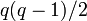# Element structure of projective general linear group:PGL(2,9)

## Contents

This article gives specific information, namely, element structure, about a particular group, namely: projective general linear group:PGL(2,9).
View element structure of particular groups | View other specific information about projective general linear group:PGL(2,9)

This article describes the element structure of projective general linear group:PGL(2,9), which is the projective general linear group of degree two over field:F9.

Note that this group is not isomorphic to symmetric group:S6, even though it has a subgroup of index two that is isomorphic to alternating group:A6.

## Conjugacy class structure

There is a total of$11$ conjugacy classes.

Nature of conjugacy class upstairs in$GL_2$ Eigenvalues Characteristic polynomial Minimal polynomial Size of conjugacy class Number of such conjugacy classes Total number of elements
Diagonalizable over field:F9 with equal diagonal entries, hence a scalar$\{ a, a \}$ where$a \in \mathbb{F}_9^\ast$$(x - a)^2$$x - a$ 1 1 1
Diagonalizable over$\mathbb{F}_{81}$, not over$\mathbb{F}_9$, eigenvalues are negatives of each other. Pair of mutually negative conjugate elements of$\mathbb{F}_{81}$. All such pairs identified.$x^2 - \mu$,$\mu$ a nonzero non-square Same as characteristic polynomial 36 1$q(q - 1)/2$
Diagonalizable over$\mathbb{F}_9$ with mutually negative diagonal entries.$\{ \lambda, - \lambda \}$, all such pairs identified.$x^2 - \lambda^2$, all identified Same as characteristic polynomial 45 1 45
Diagonalizable over$\mathbb{F}_{81}$, not over$\mathbb{F}_9$, eigenvalues are not negatives of each other. Pair of conjugate elements of$\mathbb{F}_{81}$. Each pair identified with anything obtained by multiplying both elements of it by an element of$\mathbb{F}_9$.$x^2 - ax + b$,$a \ne 0$, irreducible; with identification. Same as characteristic polynomial 72 4 288
Not diagonal, has Jordan block of size two$a \in\mathbb{F}_9^\ast$ (multiplicity 2). Each conjugacy class has one representative of each type.$(x - a)^2$ Same as characteristic polynomial 80 1 80
Diagonalizable over$\mathbb{F}_9$ with distinct diagonal entries whose sum is not zero.$\lambda, \mu$ where$\lambda,\mu \in \mathbb{F}_9^\ast$ and$\lambda + \mu \ne 0$. The pairs$\{ \lambda, \mu \}$ and$\{ a\lambda, a\mu \}$ are identified.$x^2 - (\lambda + \mu)x + \lambda\mu$, again with identification. Same as characteristic polynomial. 90 3 270
Total NA NA NA NA 11 720